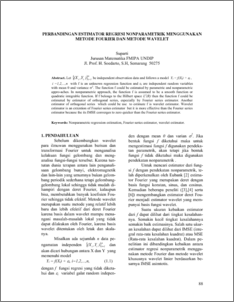# PERBANDINGAN ESTIMATOR REGRESI NONPARAMETRIK MENGGUNAKAN METODE FOURIER DAN METODE WAVELET

SUPARTI, SUPARTI (2005) PERBANDINGAN ESTIMATOR REGRESI NONPARAMETRIK MENGGUNAKAN METODE FOURIER DAN METODE WAVELET. Jurnal Matematika, 8 (3). pp. 88-94. ISSN 1410-8518Preview
PDF
167Kb

## Abstract

Let be independent observation data and follows a model Yi = f(Xi) + I , i =1,2,...,n with f is an unknown regression function and i are independent random variables with mean 0 and variance 2. The function f could be estimated by parametric and nonparametric appro-aches. In nonparametric approach, the function f is assumed to be a smooth function or quadratic integrable function. If f belongs to the Hilbert space L2(R) then the function f could be estimated by estimator of orthogonal series, especially by Fourier series estimator. Another estimator of orthogonal series which could be use to estimate f is wavelet estimator. Wavelet estimator is an extention of Fourier series estimator but it is more effective than the Fourier series estimator because the its IMSE converges to zero quicker than the Fourier series estimator.

Item Type: Article Nonparametric regression estimation, Fourier series estimator, wavelet estimator. Q Science > QA Mathematics Faculty of Science and Mathematics > Department of Mathematics 2079 INVALID USER 02 Dec 2009 15:23 23 Dec 2009 14:45

Repository Staff Only: item control page